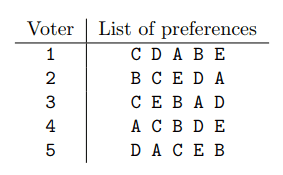### J - Jeopardized Election

##### Languages: MS C# .NET 4.7.2053,GNU G++11 5.1.0 ...

Nlogonian elections are coming up soon and there are many candidates running for President of one of the greatest nations on Earth.

The voting system used in Nlogonia is quite out of the ordinary. Each person votes by making a list of all candidates, in order of preferences of the voter. This means that the first candidate in the list is the one whose proposals please the voter most, and the last candidate in the list is the one whose proposals please the voter least.

Suppose that there are exactly five voters $1, 2, 3, 4$ and $5$, exactly five candidates $A, B, C, D$ and $E$, and the voters voted as shown in the following table:To determine the winner, the Electoral Commission first makes a draw, called Election Ordering, which contains all the candidates in a certain order. Then each candidate is evaluated following the Election Ordering, until one of them is elected as President. For this to happen, the current evaluated candidate must be the preferred still-running candidate for more than half of the voters.

To make the election system clearer, continuing the example above, suppose that the result of the Election Ordering is $C, D, A, E$ and $B$. To determine the winner the Electoral Commission would perform the following steps:

- The first candidate evaluated is $C$. As this candidate is the preferred candidate for just two of the five voters ($1$ and $3$), then $C$ is eliminated.
- Next candidate evaluated is $D$, who is the preferred still-running candidate for only two voters ($1$ and $5$). Thus, candidate $D$ is also eliminated.
- Candidate $A$ is evaluated next. Since this candidate is the preferred still-running candidate for three of the five voters ($1$, $4$ and $5$), candidate $A$ is elected as President and the voting ends.

One of the candidates has managed to corrupt some members of the Electoral Commission, and can now decide what the result of the Election Ordering will be. Also, thanks to various social networks analysis, the candidate knows the list that each voter will vote. The only thing the candidate needs to win the election now is to figure out a proper Election Ordering. As this is not an easy task, someone from the candidate staff anonymously hired you to find an ordering that makes the candidate win. Hurry up, because the draw will occur within the next few hours.

#### Input

The first line contains two integers $C$ and $V$ ($1 \leq C, V \leq 100$, with $V$ odd), representing respectively the number of candidates and the number of voters. Candidates are identified by distinct non-empty strings of at most $10$ uppercase letters. Each of the next $V$ lines describes the vote of a voter, that is, the line contains the list of candidates in order of preference of the voter. All lists contain the same candidates, although candidates may appear in different order. After the votes there is a last line that contains a string $W$, indicating the candidate that must win.

#### Output

Output a single line with the Election Ordering that makes candidate $W$ win the election, or the character “*” (asterisk) if it is not possible for $W$ to win. If more than one possible Election Ordering exists, output the lexicographically smallest one.

#### Sample test(s)

Input
5 5 C D A B E B C E D A C E B A D A C B D E D A C E B A
Output
C B D A E
Input
3 5 KATE BOB ED BOB ED KATE ED BOB KATE BOB ED KATE KATE BOB ED KATE
Output
*
Input
3 5 KATE BOB ED BOB ED KATE ED BOB KATE BOB ED KATE KATE BOB ED ED
Output
BOB ED KATE